# Frames

Functions to transform rotational frame quantities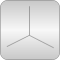# Package Contents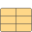Orientation Orientation object defining rotation from a frame 1 into a frame 2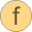orientationConstraint Return residues of orientation constraints (shall be zero)angularVelocity1 Return angular velocity resolved in frame 1 from orientation objectangularVelocity2 Return angular velocity resolved in frame 2 from orientation objectresolve1 Transform vector from frame 2 to frame 1resolve2 Transform vector from frame 1 to frame 2resolveRelative Transform vector from frame 1 to frame 2 using absolute orientation objects of frame 1 and of frame 2resolveDyade1 Transform second order tensor from frame 2 to frame 1resolveDyade2 Transform second order tensor from frame 1 to frame 2nullRotation Return orientation object that does not rotate a frameinverseRotation Return inverse orientation objectrelativeRotation Return relative orientation objectabsoluteRotation Return absolute orientation object from another absolute and a relative orientation objectplanarRotation Return orientation object of a planar rotationplanarRotationAngle Return angle of a planar rotation, given the rotation axis and the representations of a vector in frame 1 and frame 2axisRotation Return rotation object to rotate around an angle along one frame axisaxesRotations Return fixed rotation object to rotate in sequence around fixed angles along 3 axesaxesRotationsAngles Return the 3 angles to rotate in sequence around 3 axes to construct the given orientation objectsmallRotation Return rotation angles valid for a small rotation and optionally residues that should be zerofrom_nxy Return fixed orientation object from n_x and n_y vectorsfrom_nxz Return fixed orientation object from n_x and n_z vectorsfrom_T Return orientation object R from transformation matrix Tfrom_T2 Return orientation object R from transformation matrix T and its derivative der(T)from_T_inv Return orientation object R from inverse transformation matrix T_invfrom_Q Return orientation object R from quaternion orientation object Qto_T Return transformation matrix T from orientation object Rto_T_inv Return inverse transformation matrix T_inv from orientation object Rto_Q Return quaternion orientation object Q from orientation object Rto_vector Map rotation object into vectorto_exy Map rotation object into e_x and e_y vectors of frame 2, resolved in frame 1axis Return unit vector for x-, y-, or z-axis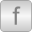Quaternions Functions to transform rotational frame quantities based on quaternions (also called Euler parameters)TransformationMatrices Functions for transformation matrices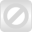Internal Internal definitions that may be removed or changed (do not use)

# Information

This information is part of the Modelica Standard Library maintained by the Modelica Association.

Package Frames contains type definitions and functions to transform rotational frame quantities. The basic idea is to hide the actual definition of an orientation in this package by providing essentially type Orientation together with functions operating on instances of this type.

#### Content

In the table below an example is given for every function definition. The used variables have the following declaration:

```   Frames.Orientation R, R1, R2, R_rel, R_inv;
Real[3,3]   T, T_inv;
Real     v1, v2, w1, w2, n_x, n_y, n_z, e, e_x, res_ori, phi;
Real     res_equal;
Real        L, angle;
```
Function/typeDescription
Orientation R; New type defining an orientation object that describes
the rotation of frame 1 into frame 2.
res_ori = orientationConstraint(R); Return the constraints between the variables of an orientation object
(shall be zero).
w1 = angularVelocity1(R); Return angular velocity resolved in frame 1 from orientation object R.
w2 = angularVelocity2(R); Return angular velocity resolved in frame 2 from orientation object R.
v1 = resolve1(R,v2); Transform vector v2 from frame 2 to frame 1.
v2 = resolve2(R,v1); Transform vector v1 from frame 1 to frame 2.
v2 = resolveRelative(v1,R1,R2); Transform vector v1 from frame 1 to frame 2 using absolute orientation objects R1 of frame 1 and R2 of frame 2.
D1 = resolveDyade1(R,D2); Transform second order tensor D2 from frame 2 to frame 1.
D2 = resolveDyade2(R,D1); Transform second order tensor D1 from frame 1 to frame 2.
R = nullRotation() Return orientation object R that does not rotate a frame.
R_inv = inverseRotation(R); Return inverse orientation object.
R_rel = relativeRotation(R1,R2); Return relative orientation object from two absolute orientation objects.
R2 = absoluteRotation(R1,R_rel); Return absolute orientation object from another absolute
and a relative orientation object.
R = planarRotation(e, angle, der_angle); Return orientation object of a planar rotation.
angle = planarRotationAngle(e, v1, v2); Return angle of a planar rotation, given the rotation axis
and the representations of a vector in frame 1 and frame 2.
R = axisRotation(axis, angle, der_angle); Return orientation object R to rotate around angle along axis of frame 1.
R = axesRotations(sequence, angles, der_angles); Return rotation object to rotate in sequence around 3 axes. Example:
R = axesRotations({1,2,3},{pi/2,pi/4,-pi}, zeros(3));
angles = axesRotationsAngles(R, sequence); Return the 3 angles to rotate in sequence around 3 axes to
construct the given orientation object.
phi = smallRotation(R); Return rotation angles phi valid for a small rotation R.
R = from_nxy(n_x, n_y); Return orientation object from n_x and n_y vectors.
R = from_nxz(n_x, n_z); Return orientation object from n_x and n_z vectors.
R = from_T(T,w); Return orientation object R from transformation matrix T and its angular velocity w.
R = from_T2(T,der(T)); Return orientation object R from transformation matrix T and its derivative der(T).
R = from_T_inv(T_inv,w); Return orientation object R from inverse transformation matrix T_inv and its angular velocity w.
R = from_Q(Q,w); Return orientation object R from quaternion orientation object Q and its angular velocity w.
T = to_T(R); Return transformation matrix T from orientation object R.
T_inv = to_T_inv(R); Return inverse transformation matrix T_inv from orientation object R.
Q = to_Q(R); Return quaternion orientation object Q from orientation object R.
exy = to_exy(R); Return [e_x, e_y] matrix of an orientation object R,
with e_x and e_y vectors of frame 2, resolved in frame 1.
L = length(n_x); Return length L of a vector n_x.
e_x = normalize(n_x); Return normalized vector e_x of n_x such that length of e_x is one.
e = axis(i); Return unit vector e directed along axis i
Quaternions Package with functions to transform rotational frame quantities based on quaternions (also called Euler parameters).
TransformationMatrices Package with functions to transform rotational frame quantities based on transformation matrices.